# Parent Function Transformations Linear Parent Function Transformation Function

• Slides: 27Parent Function TransformationsLinear Parent Function Transformation Function Translation (b) Move down 4 unit b moves up or down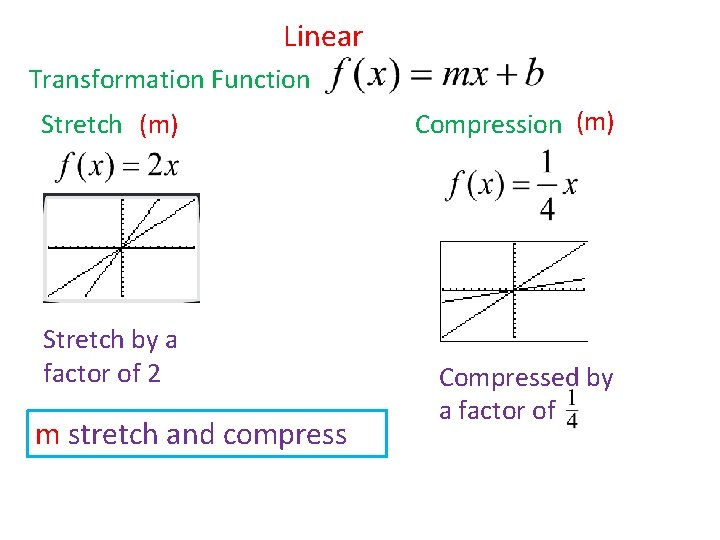Linear Transformation Function Stretch (m) Stretch by a factor of 2 m stretch and compress Compression (m) Compressed by a factor ofLinear Transformation Function reflection (m) Reflect over the x-axis m reflection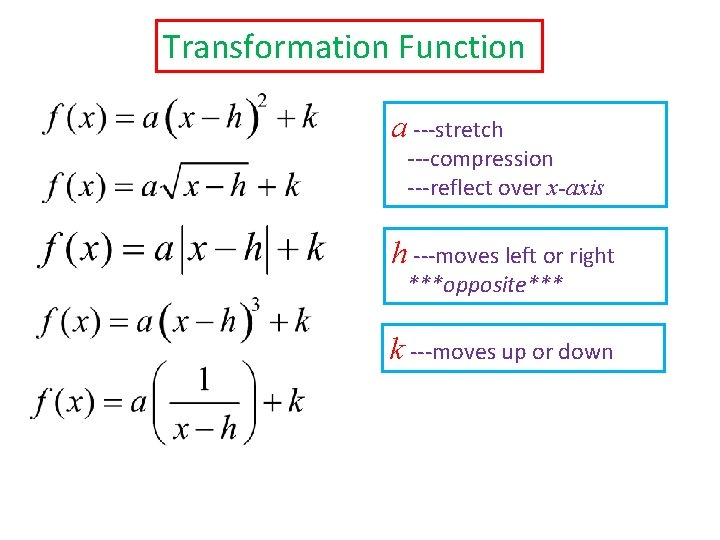Transformation Function a ---stretch ---compression ---reflect over x-axis h ---moves left or right ***opposite*** k ---moves up or downQuadratic Horizontal Translation (h) Move left 2 units Move right 4 units h moves left or right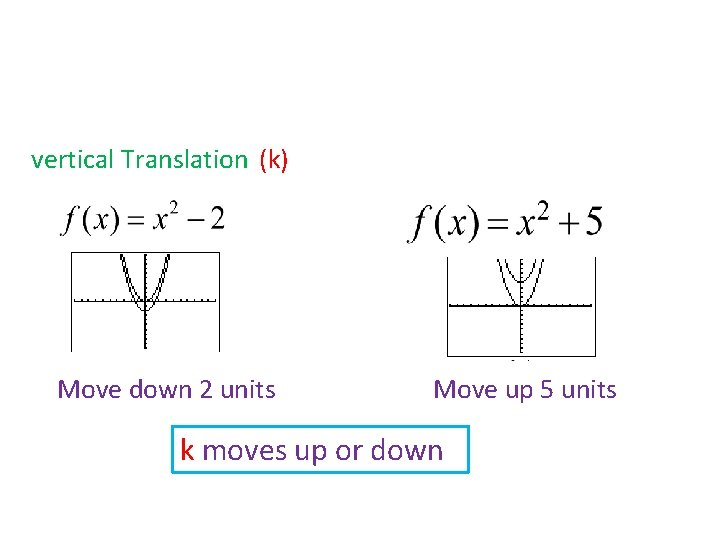vertical Translation (k) Move down 2 units Move up 5 units k moves up or downQuadratic stretch (a) Stretch by a factor of 2 Compression (a) compressed by a factor of reflection (a) Reflect over the x-axis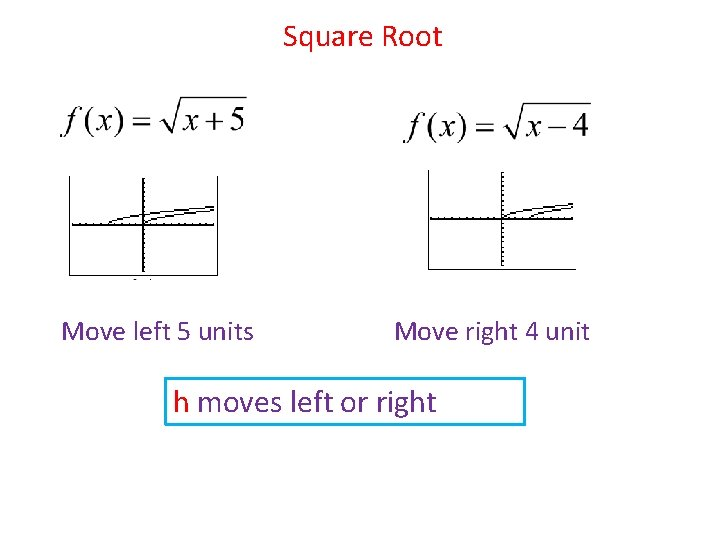Square Root Move left 5 units Move right 4 unit h moves left or rightMove up 3 units Move down 8 units k moves up or downStretch by a factor of 2 compressed by a factor of Reflect over the x-axis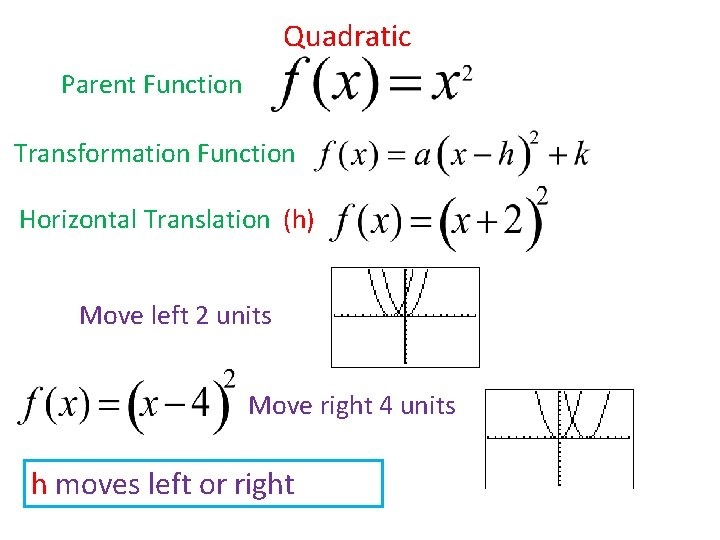Quadratic Parent Function Transformation Function Horizontal Translation (h) Move left 2 units Move right 4 units h moves left or right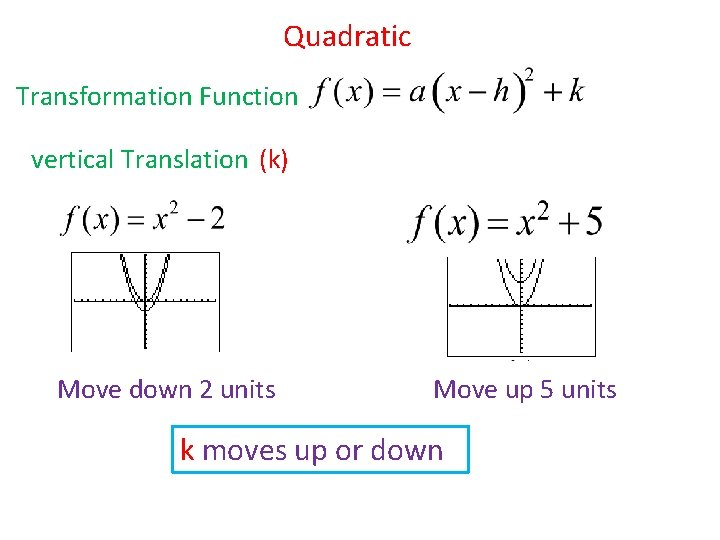Quadratic Transformation Function vertical Translation (k) Move down 2 units Move up 5 units k moves up or down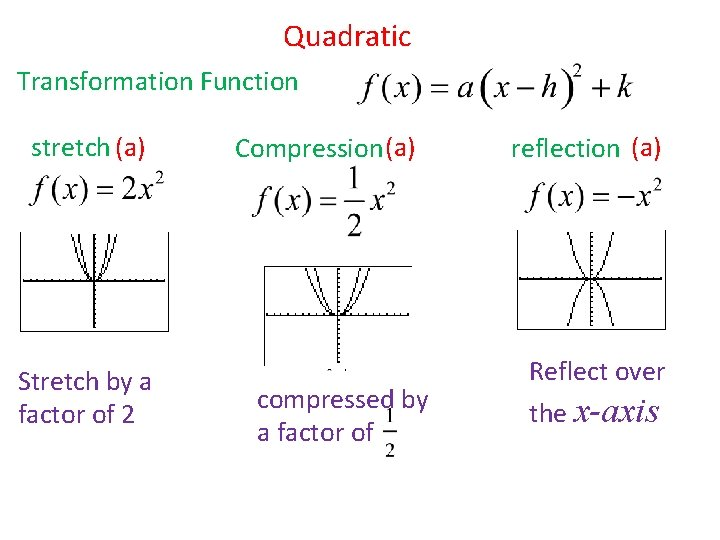Quadratic Transformation Function stretch (a) Stretch by a factor of 2 Compression (a) compressed by a factor of reflection (a) Reflect over the x-axis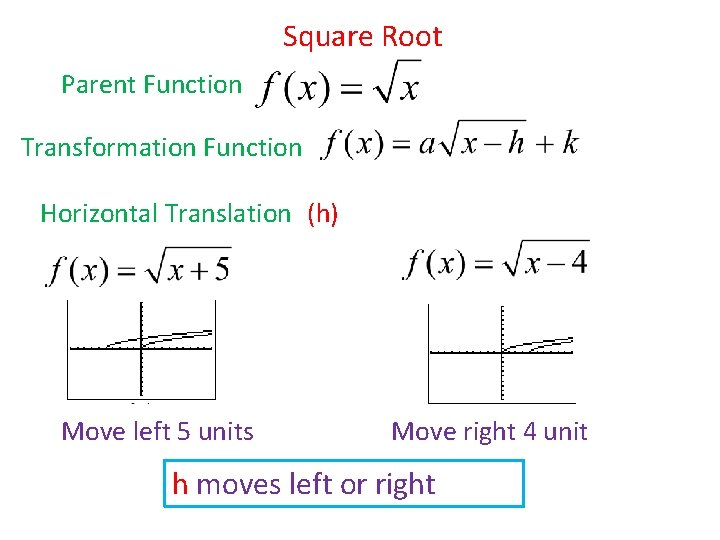Square Root Parent Function Transformation Function Horizontal Translation (h) Move left 5 units Move right 4 unit h moves left or right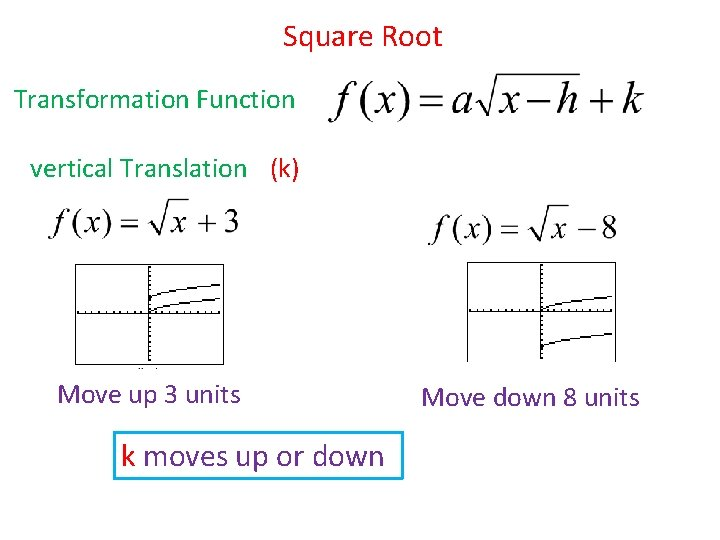Square Root Transformation Function vertical Translation (k) Move up 3 units k moves up or down Move down 8 units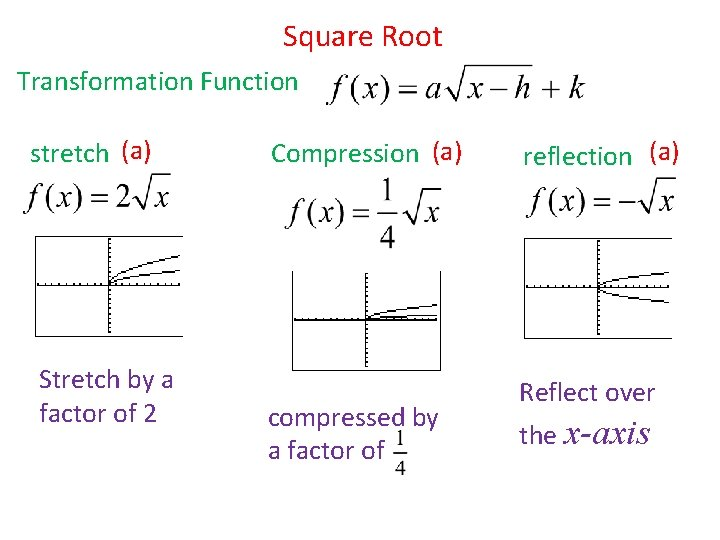Square Root Transformation Function stretch (a) Stretch by a factor of 2 Compression (a) compressed by a factor of reflection (a) Reflect over the x-axisAbsolute Value Parent Function Transformation Function Horizontal Translation (h) Move right 5 units Move left 2 unit h moves left or rightAbsolute Value Transformation Function vertical Translation (k) Move up 2 units Move down 4 units k moves up or downAbsolute Value Transformation Function stretch (a) Compression (a) reflection (a) Stretch by a factor of 2 compressed by a factor of Reflect over the x-axisCubic Parent Function Transformation Function Horizontal Translation (h) Move right 4 units Move left 2 units h moves left or right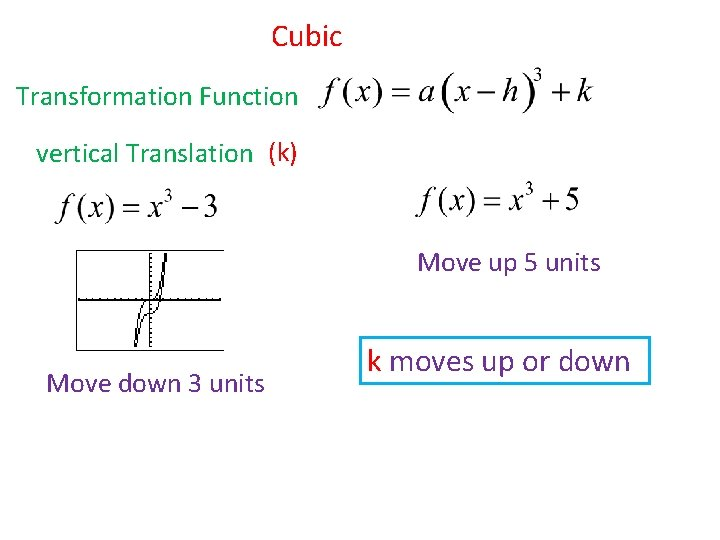Cubic Transformation Function vertical Translation (k) Move up 5 units Move down 3 units k moves up or downCubic Transformation Function stretch (a) Compression (a) reflection (a) Stretch by a factor of 4 compressed by a factor of Reflect over the x-axis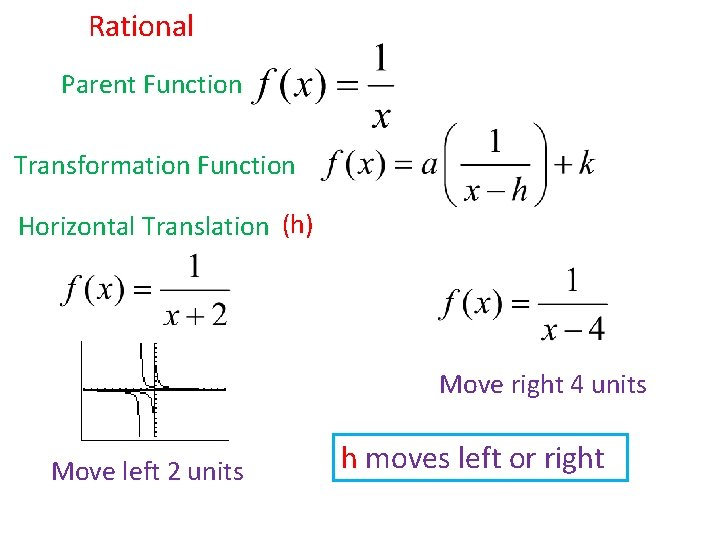Rational Parent Function Transformation Function Horizontal Translation (h) Move right 4 units Move left 2 units h moves left or rightRational Transformation Function vertical Translation (k) Move down 5 units Move up 3 units k moves up or down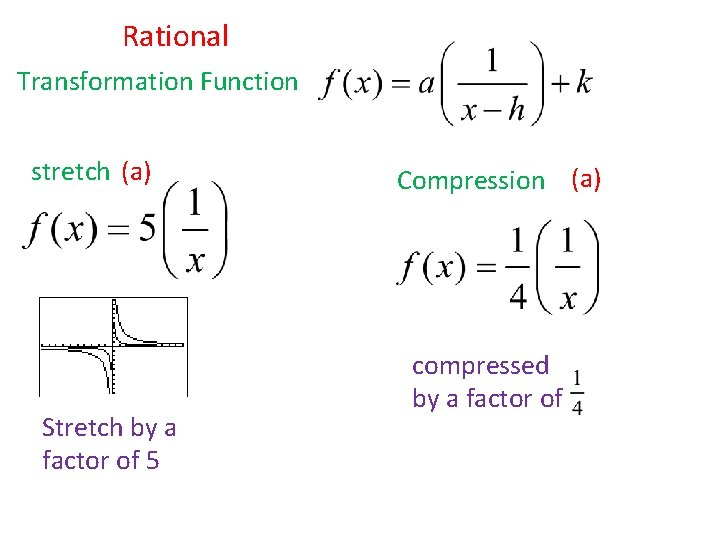Rational Transformation Function stretch (a) Stretch by a factor of 5 Compression (a) compressed by a factor ofRational Transformation Function reflection (a) Reflect over the x-axis